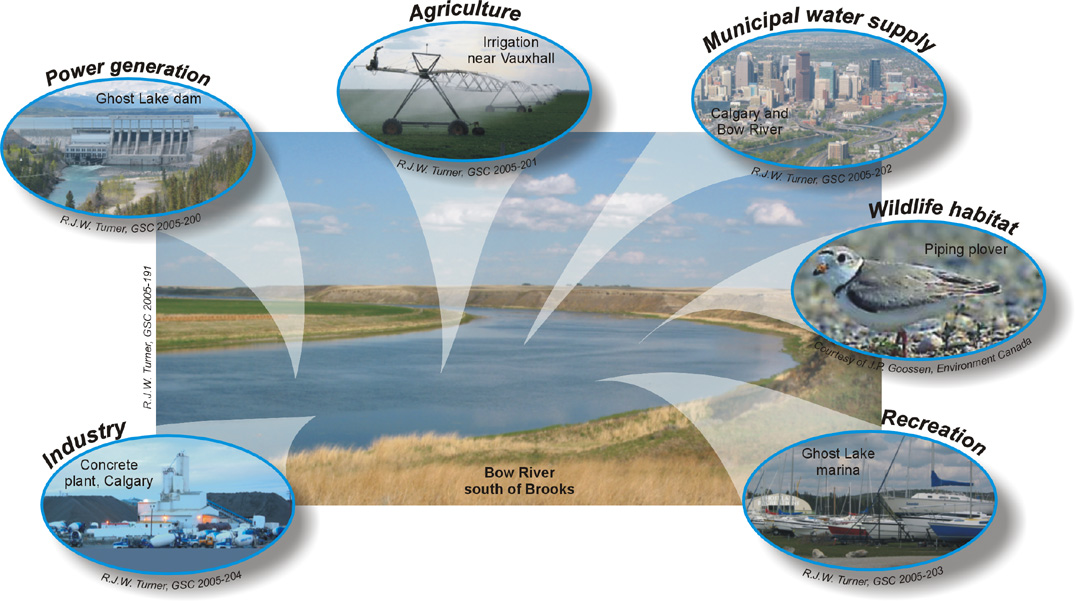Feb 26, 2016

Feb 26, 2016

Feb 26, 2016

# Methods of Estimation of Consumptive Use of WaterConsumptive water use is water removed from available supplies without return to a water resource system (e.g., water used in manufacturing, agriculture, and food preparation that is not returned to a stream, river, or water treatment plant).

To measure or estimation the consumptive use there are three main methods:

1. Direct Methods/Field Methods
2. Empirical Methods
3. Pan evaporation method

## 1. Direct Methods:

In this method field observations are made and physical model is used for this purpose. This includes,

1. Vapour Transfer Method/Soil Moisture Studies
2. Field Plot Method
3. Tanks and Lysimeter
4. Integration Method/Summation Method
5. Irrigation Method
6. Inflow Outflow Method

### 1.1 Vapour Transfer Method:

In this method of estimation of water consumptive use, soil moisture measurements are taken before and after each irrigation. The quantity of water extracted per day from soil is computed for each period. A curve is drawn by plotting the rate of use against time and from this curve, the seasonal use can be estimated. This method is suitable in those areas where soil is fairly uniform and ground water is deep enough so that it does not affect the fluctuations in the soil moisture within the root zone of the soil.

It is expressed in terms of volume i.e. Acre-feet or Hectare-meter

### 1.2 Field Plot Method:

We select a representative plot of area and the accuracy depends upon the representativeness of plot (cropping intensity, exposure etc).It replicates the conditions of an actual sample field (field plot). Less seepage should be there.

Inflow + Rain + Outflow = Evapotranspiration

The drawback in this method is that lateral movement of water takes place although more representative to field condition. Also some correction has to be applied for deep percolation as it cannot be ascertained in the field.

### 1.3 Tanks and Lysimeter:

In this method of measurement of consumptive use of water, a watertight tank of cylindrical shape having diameter 2m and depth about 3m is placed vertically on the ground. The tank is filled with sample of soil. The bottom of the tank consists of a sand layer and a pan for collecting the surplus water. The plants grown in the Lysimeter should be the same as in the surrounding field. The consumptive use of water is estimated by measuring the amount of water required for the satisfactory growth of the plants within the tanks. Consumptive use of water is given by,

Cu = Wa - Wd

Where,

Cu = Consuptive use of water
Wa = Water Applied
Wd = Water drained off

Lysimeter studies are time consuming and expensive. Methods 1 and 2 are the more reliable methods as compare to this method.

### 1.4 Integration Method:

In this method, it is necessary to know the division of total area, i.e. under irrigated crops, natural native vegetation area, water surface area and bare land area. In this method, annual consumptive use for the whole area is found in terms of volume. It is expressed in Acre feet or Hectare meter.

Mathematically,

Total Evapotranspiration = Total consumptive usex

Total Area Annual Consumptive Use = Total Evapotranspiration = A+B+C+D Where,
A = Unit consumptive use for each cropxits area
B = Unit consumptive use of native vegetation xits area
C = Water surface evaporationxits area
D = Bare land evaporationxits area

### 1.5 Irrigation Method:

In this method, unit consumption is multiplied by some factor. The multiplication values depend upon the type of crops in certain area. This method requires an Engineer judgment as these factors are to be investigated by the Engineers of certain area.

### 1.6 Inflow Outflow Method:

In this method annual consumptive use is found for large areas. If U is the valley consumptive use its value is given by,

U = (I+P) + (Gs - Ge) - R

Where,

U = Valley consumptive use (in acre feet or hectare meter)
I = Total inflow during a year
P = Yearly precipitation on valley floor
Gs = Ground Storage at the beginning of the year
Ge = Ground Storage at the end of the year
R = Yearly Outflow

All the above volumes are measured in acre-feet or hectare-meter.

## 2. Empirical Methods:

Empirical equations are given for the estimation of water requirement. These are,

### 2.1 Lowry Johnson Method:

The equation for this method is,

U = 0.0015 H + 0.9 (Over specified)

U = Consumptive Use
H = Accumulated degree days during the growing season computed from maximum temperature above 32 °F

### 2.2 Penman Equation:

According to this method,

U = ET = AH + 0.27 EaA - 0.27

ET = Evapotranspiration or consumptive use in mm Ea = Evaporation (mm/day)
H = Daily head budget at surface (mm/day)
H is a function of radiation, sunshine hours, wind speed, vapour pressure and other climatic factors.
A = Slope of saturated vapour pressure curve of air at absolute temperature in °F

### 2.3 Hargreave’s Method:

It is a very simple method. According to this method,

Cu = KEp

Where,

Cu = Consumptive Use coefficient (varies from crop to crop)
Ep = Evapotranspiration K = Coefficient